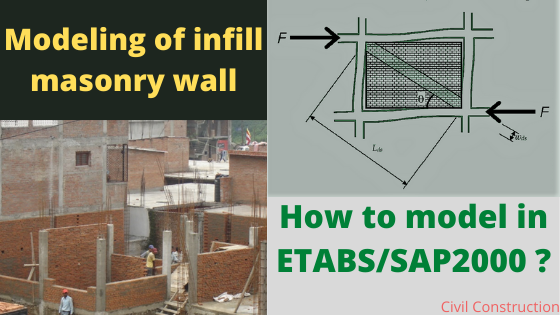Masonry wall refers to the material crafted by a mason which may be brickwork or blockwork. Brick or block are normally in cuboidal shape which can be easily lifted. If the reinforcement are used in this types of masonry wall, then these wall are termed as reinforced-masonry wall, otherwise unreinforced masonry wall. Here we discuss about the modeling of infill masonry wall (unreinforced) . Infill refers that the wall is built in between the beam or columns.

In framed structure, the space in between the column is filled with the brick or concrete block wall with cement mortar. And the construction is for the security, privacy and for partition. The unreinforced infill-masonry wall is termed as non-load bearing member in framed structure. But in actual, the masonry wall also contribute in load transfer if the connection with the structural member (beam, column) is perfect.

The reason of not considering the infill-masonry wall as the structural member in framed structure is due to its low tensile strength. And even in moderate lateral force, normal diagonal cracks to heavy destruction occurs in masonry wall.

In structural design/analysis software like ETABS, SAP2000 or STAAD PRO, the structural member are only modeled neglecting the presence of infill masonry walls. Yes, equivalent weight of masonry wall is applied in the beam during load assigning. And also it gives satisfactory result during static condition. But such ignorance may cause huge effects during seismic activities.

As mentioned in Code IS 1893 (part 1): 2016 Clause 7.9,

RC Framed building with unreinforced masonry infill walls:

1. In RC building with moment resisting frames and unreinforced masonry infill walls, variation of storey stiffness and strength shall be examined along the height of the building considering in-plane stiffness and strength of URM infill walls. If storey stiffness and strength variation along the height of the building renders it to be regular as per table 6, the irregularity should be corrected in seismic zones III, IV and V.

2. The estimation of in-plane stiffness and strength of URM infill walls shall be based on provision here under.

## Modeling of URM infill masonry wall:

URM infill walls shall be modeled by using equivalent diagonal struts as below:

1. Ends of diagonals struts shall be considered to be pin-jointed to RC frame;
2. For URM infill walls without any opening, width wds of equivalent diagonal strut shall be taken as :

wds =0.175αh-0.4 Lds

where,

αh = h⁴√(Em * t * sin2Ѳ / 4Ef * Ic *h)

where Em and Ef are the moduli of elasticity of the materials of the URM infills and RC MRF,

Ic the moment of inertia of the adjoining column, t thickness of infill walls and Ө the angle of the diagonal struts with the horizontal;

3. For URM infill walls with openings, no reduction in struts is required; and

4. Thickness of equivalent strut shall be taken as thickness t of original URM infill walls, provided h/t < 12 and l/t < 12, h is the clear height of URM infill wall between the top beam and bottom floor slab, and l clear length of the URM infill wall between the vertical RC element (column, walls, or a combination thereof) between which it spans.

The modulus of elasticity Em (in MPa ) of masonry infill-wall shall be taken as :

Em = 550fm

Where fm is the compressive strength of masonry (in MPa) obtained as per IS 1905 or given by the expression:

fm = 0.433 fb0.64 fmo0.36

Where,

fb = compressive strength of brick, in MPa and

fmo = compressive strength of mortar, in MPa

Note : A simple and conservative expression of the width of equivalent strut was proposed as:

wds = 0.25 dm

source: www.iitk.ac.in

#### EX. Modeling of infill masonry wall constructed with AAC block

The modulus of elasticity Em (in MPa ) of masonry infill-wall shall be taken as :

Em = 550fm

Where fm is the compressive strength of masonry (in MPa) obtained as per IS 1905 or given by the expression:

fm = 0.433 fb0.64 fmo0.36

fb = compressive strength of brick, in MPa

= 3-4.5 N/mm2 (AAC block)

Take fb =3.5N/mm2

fmo = compressive strength of mortar, in MPa

= 10N/mm(M10)

On substituting,

fm = 0.433 3.50.64 100.36

= 2.2115 N/mm2

And Em = 550fm =550*2.2115

= 1216.3 MPa

For URM infill walls without any opening, width wds of equivalent diagonal strut shall be taken as :

wds =0.175αh-0.4 Lds

where,

αh = h⁴√(Em * t * sin2Ѳ / 4Ef * Ic *h)

where,

Ef = the moduli of elasticity of the materials of the RC MRF

= 5000 √fck

= 5000*√30

= 27386.13 MPa

Ic = moment of inertia of adjoining column

= b d3 / 12

= 800 * 8003 /12

= 3.4133 * 1010 mm4

t = thickness of masonry infill wall

= 200mm (outer) and 150mm (inner)

h = clear height of URM infill wall between the top beam and bottom floor slab

= 3.66 – 0.4 =3.26m = 3260mm

or = 4.27 – 0.4 =3.87m = 3870mm

and Ө the angle of the diagonal struts with the horizontal

Ө = tan(V/H)

For x-axis,

1.  floor height = 3.66m

Ө = tan(V/H)

= tan(3.66/7.62)

=25.65⁰

αh = h⁴√(Em * t * sin2Ѳ / 4Ef * Ic *h)

= 3260*⁴√((1216.3 * 200 * sin 2*25.65) / (4 *27386.13 * 3.1413 * 1010 * 3260))= 1.1485

wds =0.175 αh-0.4Lds

Lds = length of equivalent diagonal struts

= (V2+H2)0.5

= (3.662 + 7.622)0.5

= 8.4534 m

wds =0.175Lds

= 0.175 *1.1485-0.4 *8453.4

= 1449.3 mm

•  floor height = 4.27m

Ө = tan(V/H)

= tan(4.27/7.62)

=29.26⁰

αh = h= 3870*= 1.339

wds =0.175Lds

Lds = length of equivalent diagonal struts

= (V2+H2)0.5

= (4.272 + 7.622)0.5

= 8.7348 m

wds =0.175Lds

= 0.175 *1.339-0.4 *8734.8

= 1360 mm

DESIGN OF SHEAR WALL

DESIGN OF BASEMENT WALL

1.Md. Rifat Bin Ahmed Majumdar says:
2.sheep foot roller says: